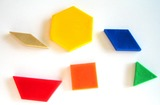Updating search results...

# 23 Results

View
Selected filters:
• sortUnrestricted Use
CC BY
Rating
0.0 stars

This resource is from Tools4NCTeachers.In this introductory lesson, students will explore and sort two-dimensional shapes and describe how they were sorted using defining and non-defining attributes.&nbsp; This lesson could be modified by exploring and sorting three-dimensional shapes.&nbsp; Remix this lesson to include extension ideas.

Subject:
Mathematics
Material Type:
Activity/Lab
Lesson
Lesson Plan
Author:
DAWNE COKER
06/12/2020Unrestricted Use
CC BY
Rating
0.0 stars

This resource is from Tools4NCTeachers.In this lesson, students use their understanding of word problems to sort problems based on type.&nbsp; The focus for students in this lesson shifts from solving word problems to analyzing word problem structures in order to group like problems.&nbsp; The purpose is to help students understand and visualize problems so they will know how to write equations that match and know what operation is needed to solve.&nbsp; This lesson focuses on sorting addition and subtraction word problems with a specific focus on Take Apart and Put Together types.&nbsp; The lesson, however, can be adjusted to use with any problem types you notice students need more experience with. &nbsp;You could also adjust the number size in the problems to use this lesson at different times in the year.&nbsp;

Subject:
Mathematics
Material Type:
Lesson
Lesson Plan
Author:
DAWNE COKER
06/14/2020Unrestricted Use
CC BY
Rating
0.0 stars

This resource is from Tools4NCTeachers.&nbsp;&nbsp;This lesson will involve interviewing classmates about where they would like to spend their summer vacation.&nbsp; Their choices will be the beach, mountains, time with a relative, or camping.&nbsp; They will create a picture graph to represent the data collected.This lesson comes with printable materials and clipart for creating a class graph.

Subject:
Mathematics
Material Type:
Activity/Lab
Lesson
Lesson Plan
Author:
DAWNE COKER